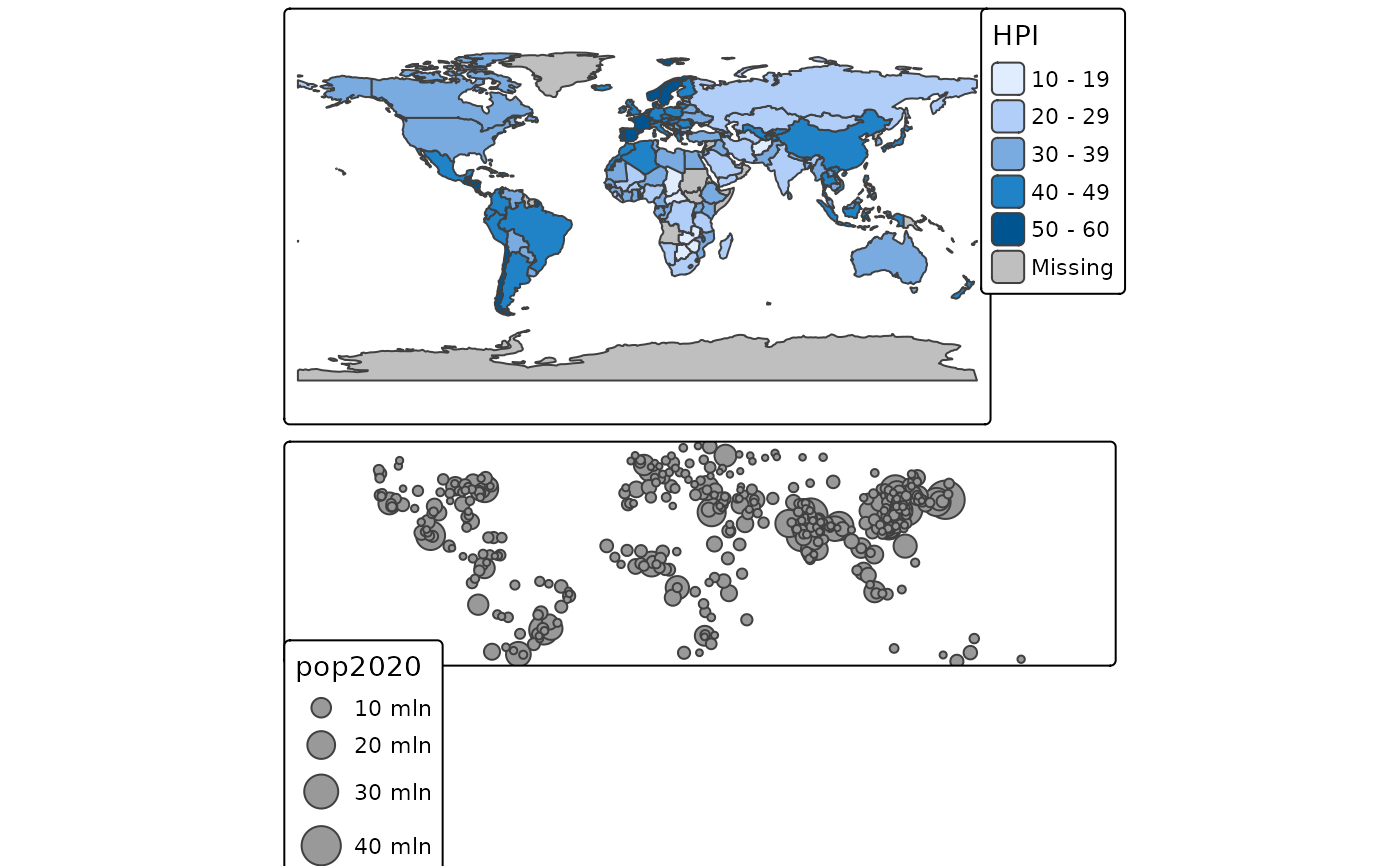Arrange small multiples in a grid layout. Normally, small multiples are created by specifying multiple variables for one aesthetic or by specifying the by argument (see tm_facets()). This function can be used to arrange custom small multiples in a grid layout.

## Usage

tmap_arrange(
...,
ncol = NA,
nrow = NA,
widths = NA,
heights = NA,
sync = FALSE,
asp = 0,
outer.margins = 0.02
)

knit_print.tmap_arrange(x, ..., options = NULL)

# S3 method for tmap_arrange
print(x, knit = FALSE, ..., options = NULL)

## Arguments

...

tmap objects or one list of tmap objects. The number of multiples that can be plot is limited (see details).

ncol

number of columns

nrow

number of rows

widths

vector of column widths. It should add up to 1 and the length should be equal to ncol.

heights

vector of row heights. It should add up to 1 and the length should be equal to nrow.

sync

logical. Should the navigation in view mode (zooming and panning) be synchronized? By default FALSE.

asp

aspect ratio. The aspect ratio of each map. Normally, this is controlled by the asp argument from tm_layout() (also a tmap option). This argument will overwrite it, unless set to NULL. The default value for asp is 0, which means that the aspect ratio is adjusted to the size of the device divided by the number of columns and rows. When asp is set to NA, which is also the default value for tm_layout(), the aspect ratio will be adjusted to the used shapes.

outer.margins

outer.margins, numeric vector four or a single value. If defines the outer margins for each multiple. If will overwrite the outer.margins argument from tm_layout(), unless set to NULL.

x

a tmap_arrange object (returned from tmap_arrange()).

options

options passed on to knitr::knit_print()

knit

should knitr::knit_print() be enabled, or the normal base::print() function?

## Details

The global option tmap.limits controls the limit of the number of facets that are plotted. By default, tmap_options(tmap.limits = c(facets.view=4, facets.plot=64)). The maximum number of interactive facets is set to four since otherwise it may become very slow.

## Examples

tm1 = tm_shape(World) + tm_polygons("HPI")
tm2 = tm_shape(metro) + tm_bubbles(size = "pop2020")

tmap_arrange(tm1, tm2)